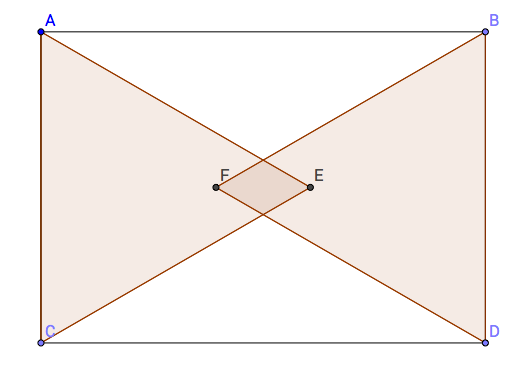# Yay for 2014! #7

Geometry Level 4Let $ABDC$ be a rectangle, as shown above, such that $AB = 20$ and $AC = 14.$ Points $E$ and $F$ are located in the interior of $ABDC$ such that the triangles $AEC$ and $BFD$ are equilateral. The area of the intersection of these triangles can be represented by

$\frac{a\sqrt{3}}{b}- c,$

where $a, b,$ and $c$ are positive integers with $\gcd(a, b) = 1.$ Find $a+b+c.$

×

Problem Loading...

Note Loading...

Set Loading...